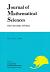## Vol. 19 (2012) No. 01Journal of Mathematical Sciences The University of Tokyo

1. Sono, Keiju
Matrix Coefficients with Minimal {\large $K$}-Types of the Spherical and Non-Spherical Principal Series Representations of {\large $SL(3,\mathbf{R})$}
Vol. 19 (2012), No. 1, Page 1--55.

Continuous Dependence for Nonlinear Schr\"odinger Equation in {\large$H^s$}
Vol. 19 (2012), No. 1, Page 57--68.

3. Berselli, Luigi C.;Romito, Marco
On Leray's Problem for Almost Periodic Flows
Vol. 19 (2012), No. 1, Page 69--130.
Addendum to On Isolated Log Canonical Singularities with Index One"